前端算法学习-算法复杂度时间复杂度

1.几种时间复杂度

• 常数阶 O(1)

// O(1)
var n = 100;
var a = 10;
console.log(a);
console.log(n);
// 总共执行4次
// 或者
for(var i=0;i<10000;i++){
console.log(i);
}
// 10000次

• 线性阶 O(n)

// O(n)
for(var i = 1; i <= n; \$i++) {
console.log(i)
}
// O(n^2)
function b(n){
for(var i = 1; i <= n; i++) {
for(var j = 1; j <= n; j++) {
console.log(j)
}
}
}
// O(2n) == O(n)
function c(n){
for(var i = 1; i <= n; i++) {
console.log(i)
}
for(var j = 1; j <= n; j++) {
console.log(j)
}
}

function a 执行的次数和n是线性关系的，所以它的时间复杂度是「O(n)」
function b 是一个嵌套循环，当n为100的情况下，里边的输出语句就会执行10000次，因此它的时间复杂度就是「O(n^2)」。比如冒泡排序的时间复杂度就是「O(n^2)」
function c 中循环不是嵌套的，而是并列的，那么它的时间复杂度应该是「O(2n)」，因为前边的常数系数我们要去掉，因此它的时间复杂度还是「O(n)」

• 对数阶 O(logn)

// O(logn)
function d(n){
var i = 1;
while(i<n)
{
i = i * 2;
}
}
// O(nlogn)
function e(n){
for(m=1; m<n; m++) {
d(n)
}
}

function e 线性对数阶O(nlogn)，就是将时间复杂度为对数阶function d的代码循环n遍的话，那么它的时间复杂度就是 n * O(logn) = O(nlogn)

• 指数阶 O(2^n)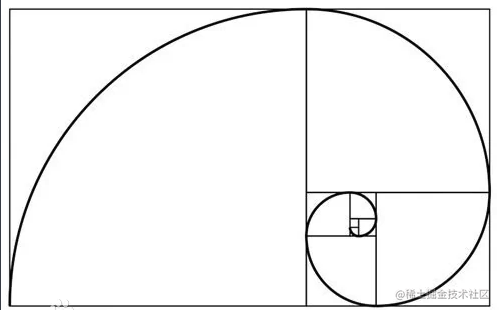斐波那契数列从 0 开始，分别是

0, 1, 1, 2, 3, 5, 8, 13, 21, 34, 55, 89, 144, 233……

function fibonacci(n){
if(n === 1 || n === 0 ) return n;
return fibonacci(n-1) + fibonacci(n-2);
}

2. 时间复杂度比较

O(1)<O(logn)<O(n)<O(nlogn)<O(n^2)<O(n^3)<O(2^n)<O(n!)<O(n^n)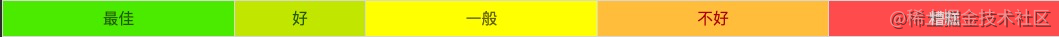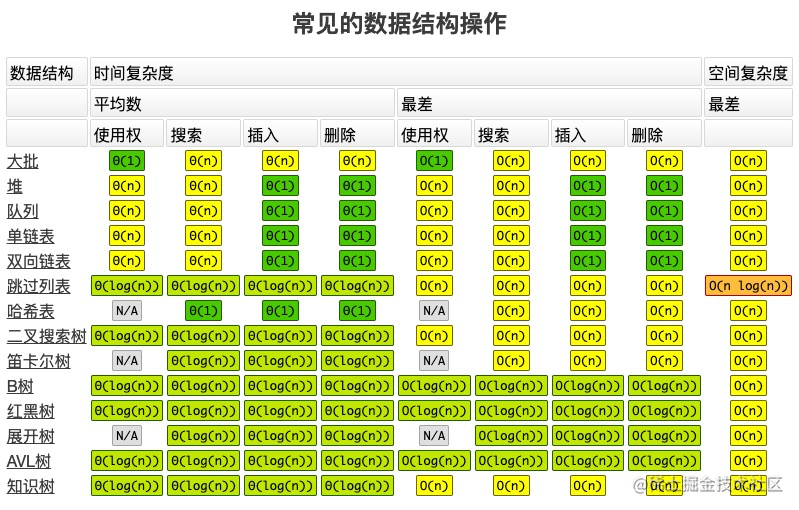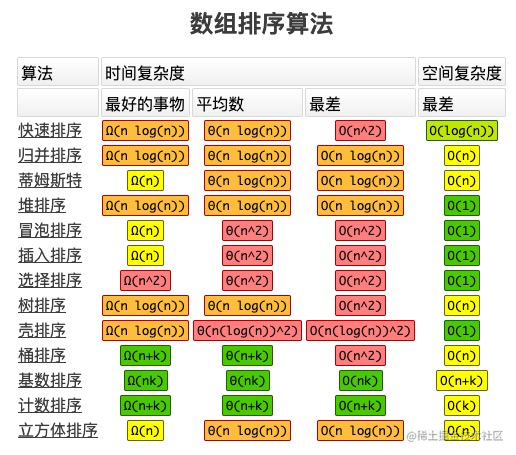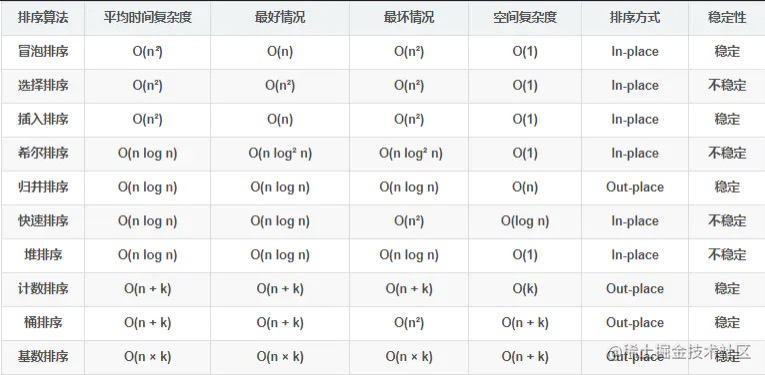3. 降低时间复杂度的应用

// 用for循环实现
function f(n){
let sum = 0
for(var i=0;i<=n;i++){
sum+=i
}
return sum
}
f(100); // 5050
function g(n){
let sum = n * (n+1)/2
return sum
}
g(100); // 5050

function f 方法的复杂度随着n的改变而增大，执行的次数和n是线性关系，时间复杂度为线性阶O(n)
function g 方法只需要执行一行代码 时间复杂度为常数阶O(1)

空间复杂度如果你的代码中开了数组，那么数组的长度基本上就是你的空间复杂度。比如你开了一个一维的数组，那么你的空间复杂度就是O(n)，如果开了一个二维的数组，数组长度是n^2，那么空间复杂度基本上就是n^2